# Selina Solutions Concise Mathematics Class 6 Chapter 8: H.C.F and L.C.M Exercise 8(C)

Selina Solutions Concise Mathematics Class 6 Chapter 8: H.C.F and L.C.M Exercise 8(C) give accurate answers in finding L.C.M by using various methods mentioned in this exercise. These solutions are designed by a set of subject matter experts to help students improve their problem-solving skills. Each and every step of Selina Solutions helps students understand in-depth and solve the textbook questions effortlessly. Students, who go through these solutions, will surely score good marks in exams. Selina Solutions Concise Mathematics Class 6 Chapter 8 H.C.F and L.C.M Exercise 8(C) PDF can be downloaded by students, from the links available below

## Selina Solutions Concise Mathematics Class 6 Chapter 8: H.C.F and L.C.M Exercise 8(C) Download PDF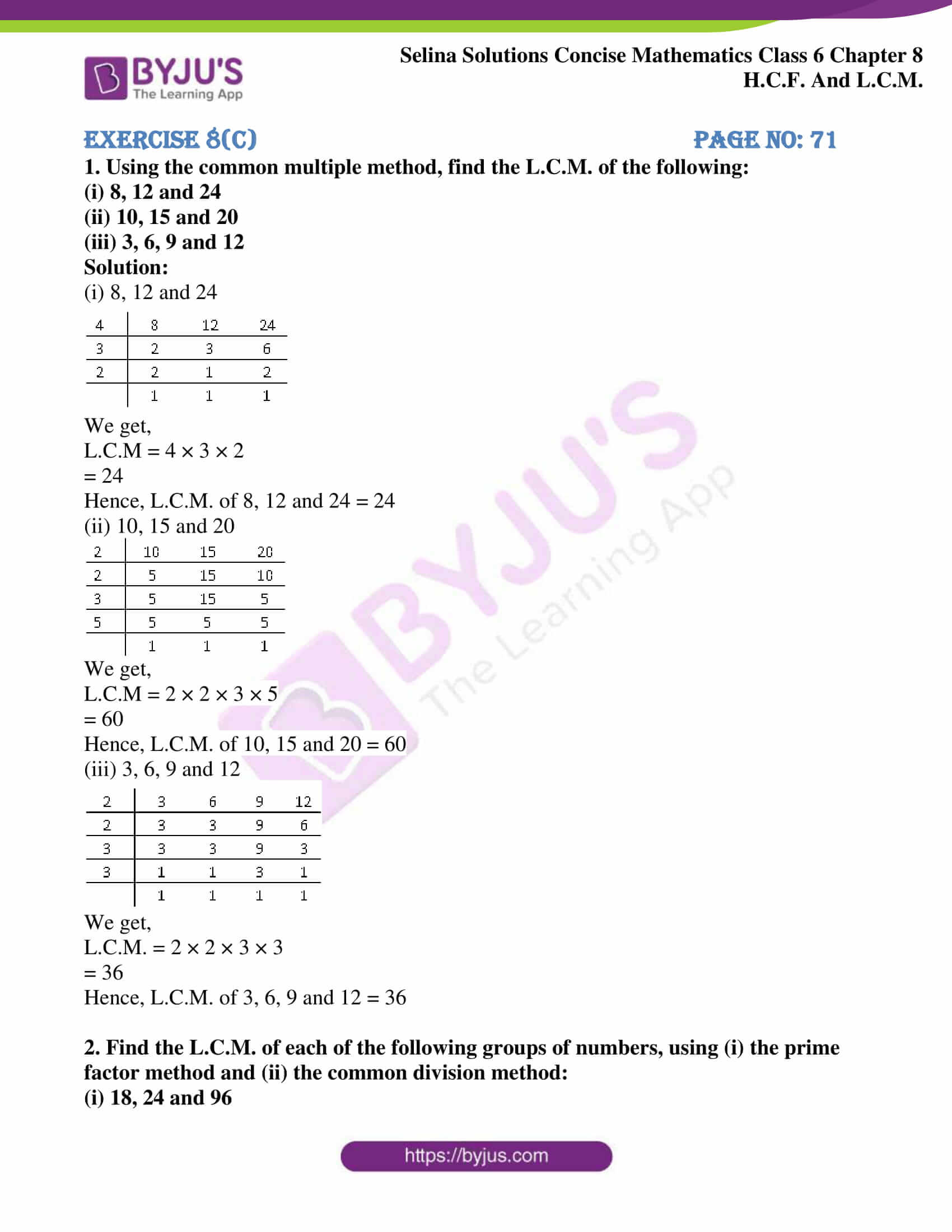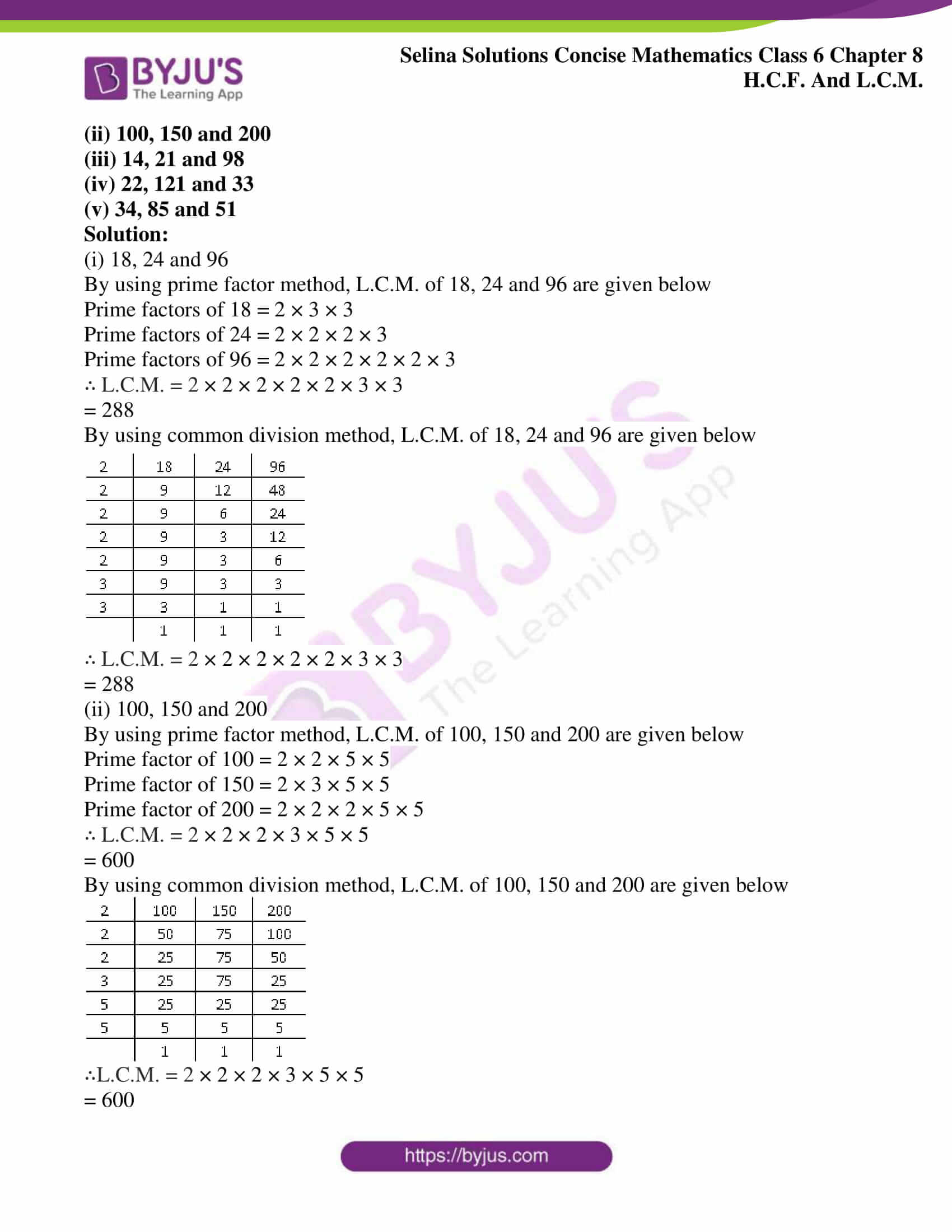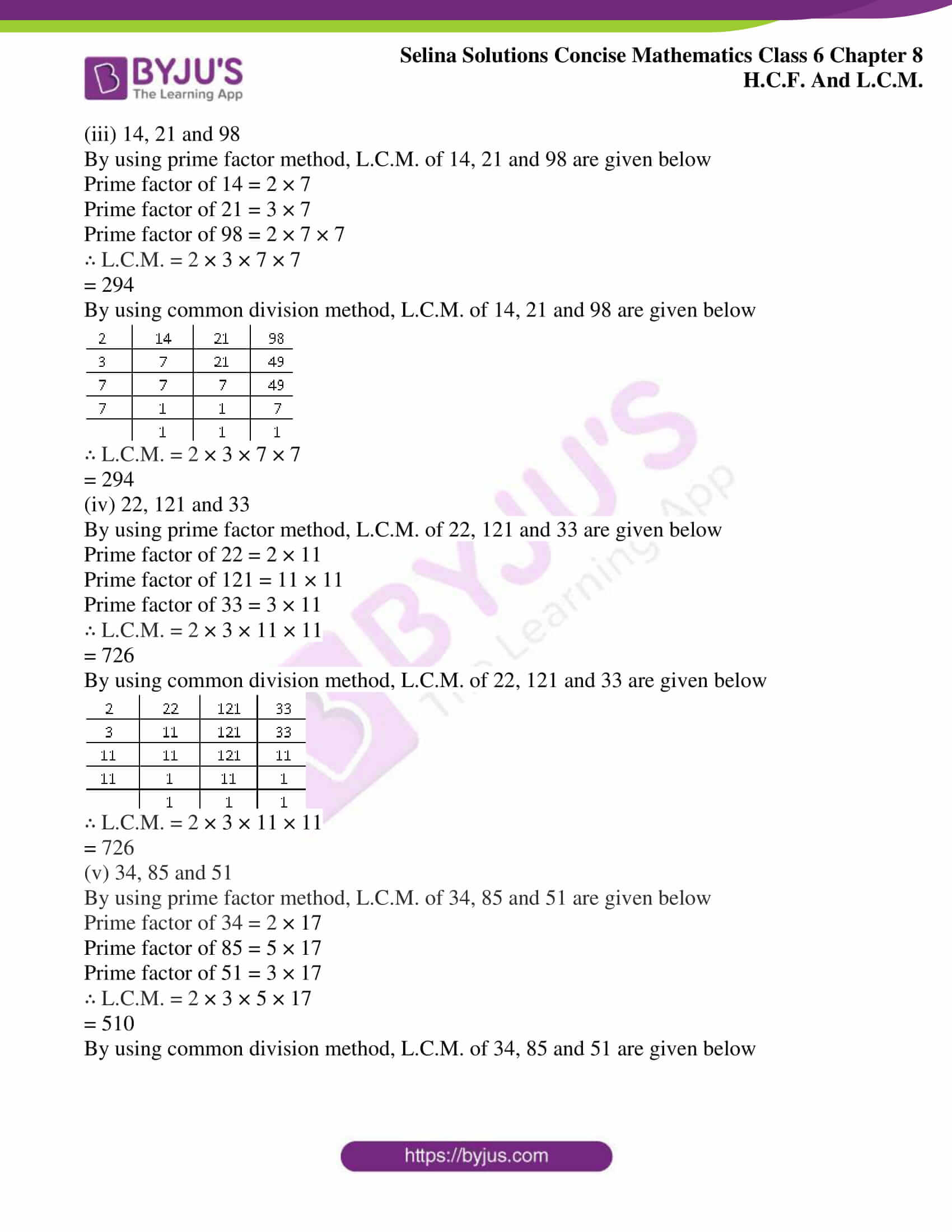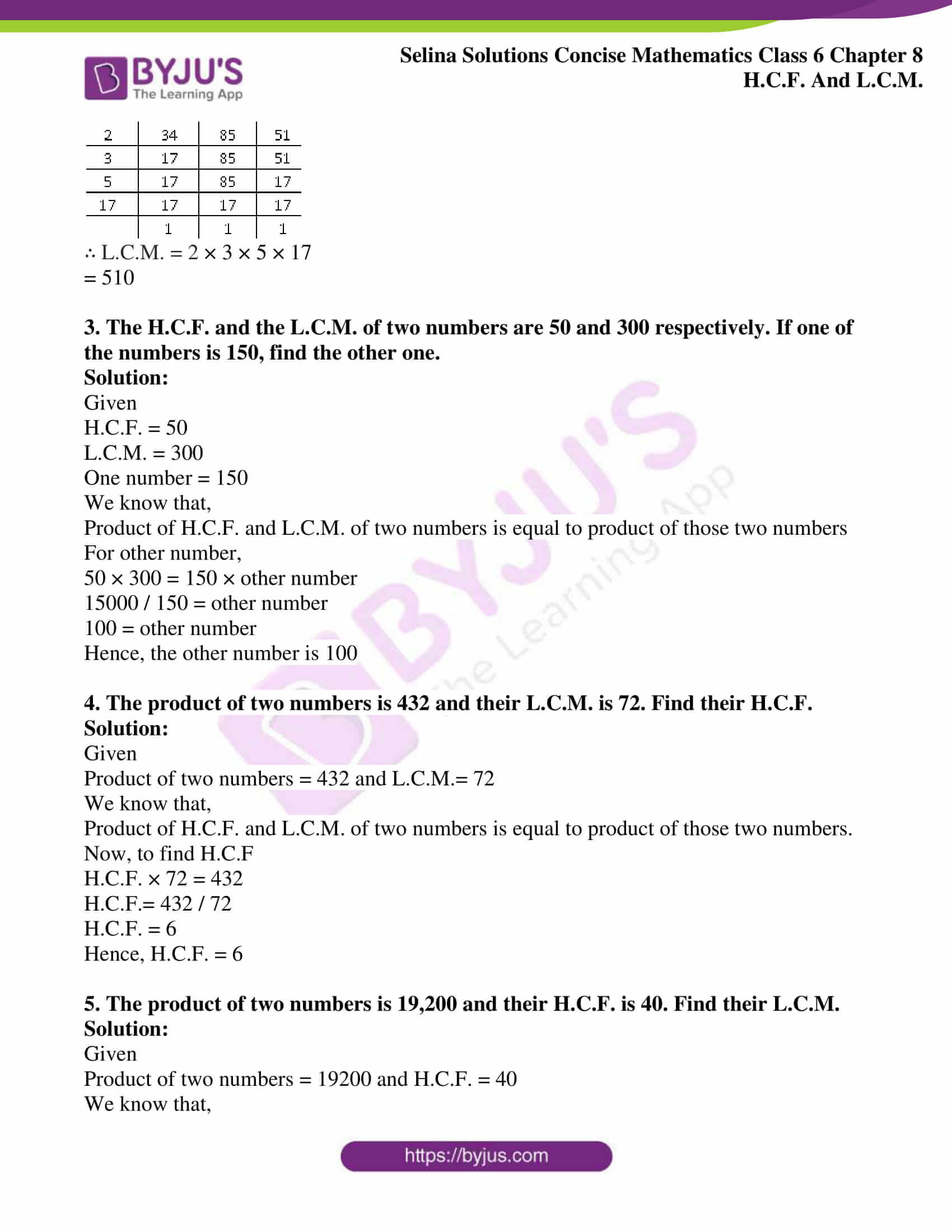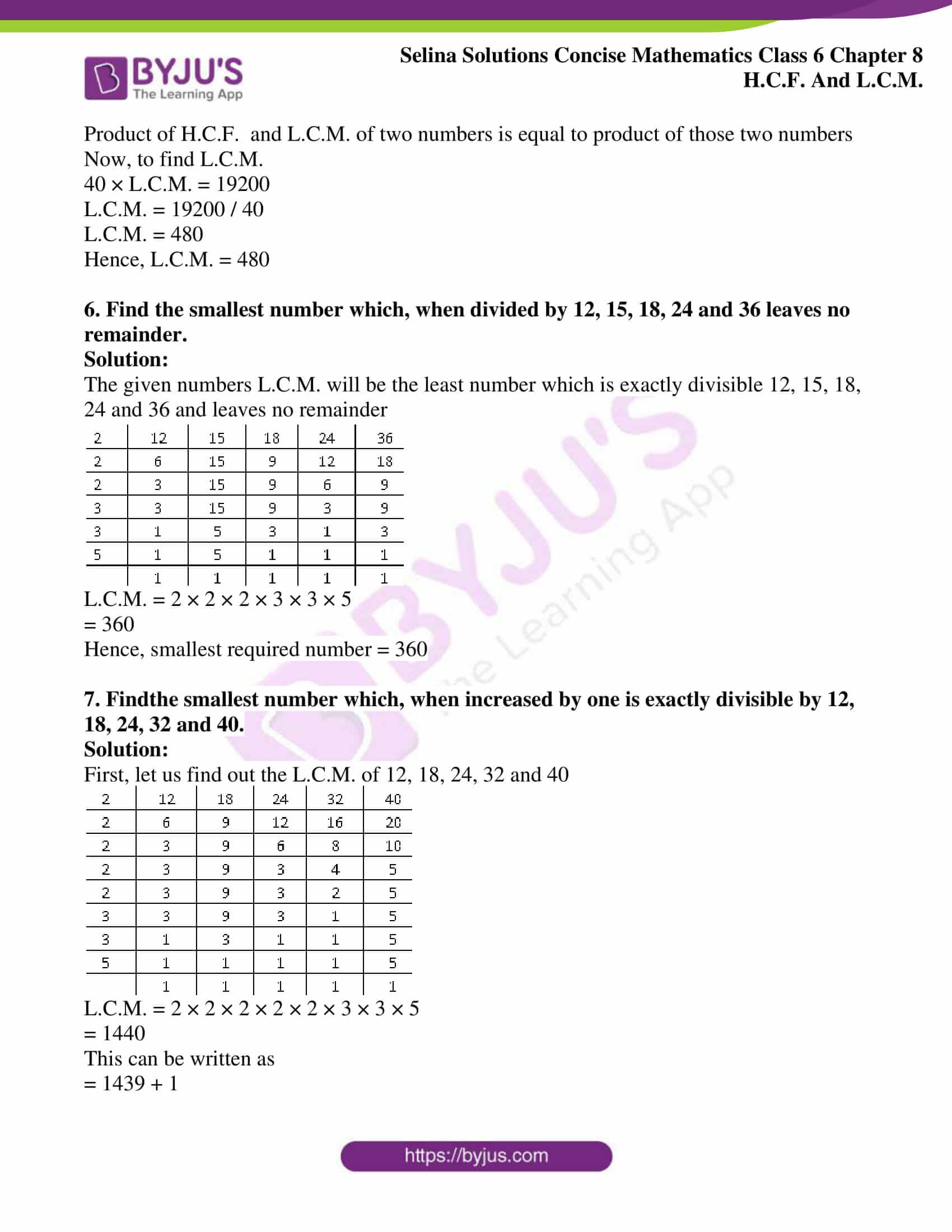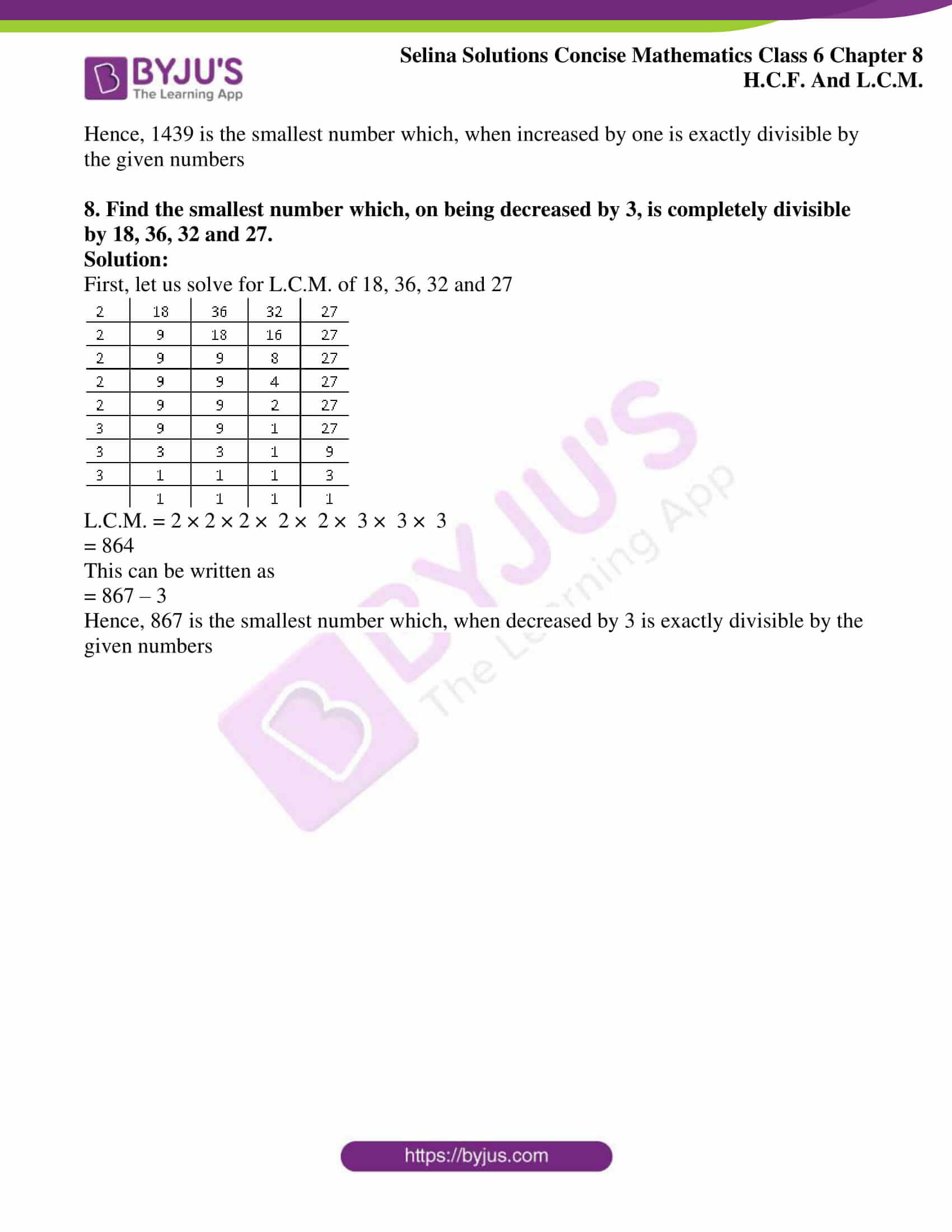### Access other exercises of Selina Solutions Concise Mathematics Class 6 Chapter 8: H.C.F and L.C.M

Exercise 8(A) Solutions

Exercise 8(B) Solutions

### Access Selina Solutions Concise Mathematics Class 6 Chapter 8: H.C.F and L.C.M Exercise 8(C)

#### Exercise 8(C) page no: 71

1. Using the common multiple method, find the L.C.M. of the following:

(i) 8, 12 and 24

(ii) 10, 15 and 20

(iii) 3, 6, 9 and 12

Solution:

(i) 8, 12 and 24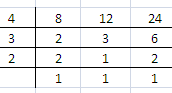We get,

L.C.M = 4 × 3 × 2

= 24

Hence, L.C.M. of 8, 12 and 24 = 24

(ii) 10, 15 and 20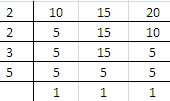We get,

L.C.M = 2 × 2 × 3 × 5

= 60

Hence, L.C.M. of 10, 15 and 20 = 60

(iii) 3, 6, 9 and 12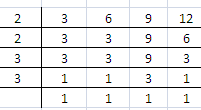We get,

L.C.M. = 2 × 2 × 3 × 3

= 36

Hence, L.C.M. of 3, 6, 9 and 12 = 36

2. Find the L.C.M. of each of the following groups of numbers, using (i) the prime factor method and (ii) the common division method:

(i) 18, 24 and 96

(ii) 100, 150 and 200

(iii) 14, 21 and 98

(iv) 22, 121 and 33

(v) 34, 85 and 51

Solution:

(i) 18, 24 and 96

By using prime factor method, L.C.M. of 18, 24 and 96 are given below

Prime factors of 18 = 2 × 3 × 3

Prime factors of 24 = 2 × 2 × 2 × 3

Prime factors of 96 = 2 × 2 × 2 × 2 × 2 × 3

∴ L.C.M. = 2 × 2 × 2 × 2 × 2 × 3 × 3

= 288

By using common division method, L.C.M. of 18, 24 and 96 are given below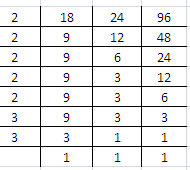∴ L.C.M. = 2 × 2 × 2 × 2 × 2 × 3 × 3

= 288

(ii) 100, 150 and 200

By using prime factor method, L.C.M. of 100, 150 and 200 are given below

Prime factor of 100 = 2 × 2 × 5 × 5

Prime factor of 150 = 2 × 3 × 5 × 5

Prime factor of 200 = 2 × 2 × 2 × 5 × 5

∴ L.C.M. = 2 × 2 × 2 × 3 × 5 × 5

= 600

By using common division method, L.C.M. of 100, 150 and 200 are given below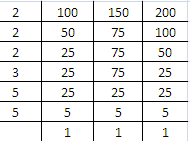∴L.C.M. = 2 × 2 × 2 × 3 × 5 × 5

= 600

(iii) 14, 21 and 98

By using prime factor method, L.C.M. of 14, 21 and 98 are given below

Prime factor of 14 = 2 × 7

Prime factor of 21 = 3 × 7

Prime factor of 98 = 2 × 7 × 7

∴ L.C.M. = 2 × 3 × 7 × 7

= 294

By using common division method, L.C.M. of 14, 21 and 98 are given below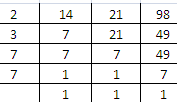∴ L.C.M. = 2 × 3 × 7 × 7

= 294

(iv) 22, 121 and 33

By using prime factor method, L.C.M. of 22, 121 and 33 are given below

Prime factor of 22 = 2 × 11

Prime factor of 121 = 11 × 11

Prime factor of 33 = 3 × 11

∴ L.C.M. = 2 × 3 × 11 × 11

= 726

By using common division method, L.C.M. of 22, 121 and 33 are given below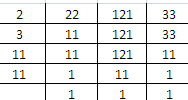∴ L.C.M. = 2 × 3 × 11 × 11

= 726

(v) 34, 85 and 51

By using prime factor method, L.C.M. of 34, 85 and 51 are given below

Prime factor of 34 = 2 × 17

Prime factor of 85 = 5 × 17

Prime factor of 51 = 3 × 17

∴ L.C.M. = 2 × 3 × 5 × 17

= 510

By using common division method, L.C.M. of 34, 85 and 51 are given below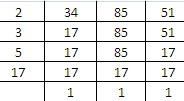∴ L.C.M. = 2 × 3 × 5 × 17

= 510

3. The H.C.F. and the L.C.M. of two numbers are 50 and 300 respectively. If one of the numbers is 150, find the other one.

Solution:

Given

H.C.F. = 50

L.C.M. = 300

One number = 150

We know that,

Product of H.C.F. and L.C.M. of two numbers is equal to product of those two numbers

For other number,

50 × 300 = 150 × other number

15000 / 150 = other number

100 = other number

Hence, the other number is 100

4. The product of two numbers is 432 and their L.C.M. is 72. Find their H.C.F.

Solution:

Given

Product of two numbers = 432 and L.C.M.= 72

We know that,

Product of H.C.F. and L.C.M. of two numbers is equal to product of those two numbers.

Now, to find H.C.F

H.C.F. × 72 = 432

H.C.F.= 432 / 72

H.C.F. = 6

Hence, H.C.F. = 6

5. The product of two numbers is 19,200 and their H.C.F. is 40. Find their L.C.M.

Solution:

Given

Product of two numbers = 19200 and H.C.F. = 40

We know that,

Product of H.C.F. and L.C.M. of two numbers is equal to product of those two numbers

Now, to find L.C.M.

40 × L.C.M. = 19200

L.C.M. = 19200 / 40

L.C.M. = 480

Hence, L.C.M. = 480

6. Find the smallest number which, when divided by 12, 15, 18, 24 and 36 leaves no remainder.

Solution:

The given numbers L.C.M. will be the least number which is exactly divisible 12, 15, 18, 24 and 36 and leaves no remainder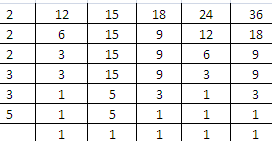L.C.M. = 2 × 2 × 2 × 3 × 3 × 5

= 360

Hence, smallest required number = 360

7. Find the smallest number which, when increased by one is exactly divisible by 12, 18, 24, 32 and 40.

Solution:

First, let us find out the L.C.M. of 12, 18, 24, 32 and 40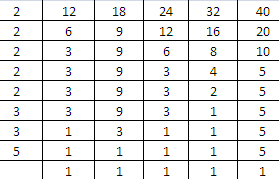L.C.M. = 2 × 2 × 2 × 2 × 2 × 3 × 3 × 5

= 1440

This can be written as

= 1439 + 1

Hence, 1439 is the smallest number which, when increased by one is exactly divisible by the given numbers

8. Find the smallest number which, on being decreased by 3, is completely divisible by 18, 36, 32 and 27.

Solution:

First, let us solve for L.C.M. of 18, 36, 32 and 27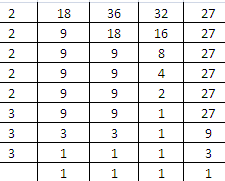L.C.M. = 2 × 2 × 2 × 2 × 2 × 3 × 3 × 3

= 864

This can be written as

= 867 – 3

Hence, 867 is the smallest number which, when decreased by 3 is exactly divisible by the given numbers